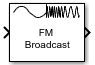Main Content

# FM Broadcast Modulator Baseband

Modulate using broadcast FM method

## Library

Modulation > Analog Baseband Modulation

•## Description

The FM Broadcast Modulator Baseband block pre-emphasizes an audio signal and modulates it onto a baseband FM signal. If you select the Stereo audio check box, the block modulates the stereo audio (LR) at the 38 kHz band, in addition to the baseband (L+R). If you select the RBDS modulation check box, the block also modulates a baseband RBDS signal at 57 kHz. For more details, see Algorithms.

## Parameters

Sample rate (Hz)

Specify the output signal sample rate as a positive real scalar.

Frequency deviation (Hz)

Specify the frequency deviation of the modulator in Hz as a positive real scalar. The system bandwidth is equal to twice the sum of the frequency deviation and the message bandwidth. FM broadcast standards specify a value of 75 kHz in the United States and 50 kHz in Europe.

Pre-emphasis filter time constant (s)

Specify the pre-emphasis highpass filter time constant as a positive real scalar. FM broadcast standards specify a value of 75 μs in the United States and 50 μs in Europe.

Sample rate of audio input signal (Hz)

Specify the input audio sample rate as a positive real scalar.

Stereo audio

Select this check box if the input signal is a stereophonic audio signal.

RBDS modulation

Select this check box to modulate a baseband RBDS signal at 57 kHz. By default, this check box is not selected.

Oversampling factor of RBDS input

Specify the number of samples per RBDS symbol as a positive integer. The RBDS sample rate is given by Oversampling factor of RBDS input × `1187.5` Hz. According to the RBDS standard, the sample rate of each bit is 1187.5 Hz.

This parameter appears when you select the RBDS modulation check box.

The default is 10.

Simulate using

Select the type of simulation to run.

• `Code generation`. Simulate model using generate C code. The first time you run a simulation, Simulink generates C code for the block. The C code is reused for subsequent simulations, as long as the model does not change. This option requires additional startup time but provides faster simulation speed than `Interpreted execution`.

• `Interpreted execution`. Simulate model using the MATLAB interpreter. This option shortens startup time but has slower simulation speed than ```Code generation```.

## Algorithms

The FM Broadcast modulator includes the functionality of the baseband FM modulator, pre-emphasis filtering, and the ability to transmit stereophonic signals. The algorithms which govern basic FM modulation and demodulation are covered in `comm.FMModulator`.

### Filtering

FM amplifies high-frequency noise and degrades the overall signal-to-noise ratio. To compensate, FM broadcasters insert a pre-emphasis filter prior to FM modulation to amplify the high-frequency content. The FM receiver has a reciprocal de-emphasis filter after the FM demodulator to attenuate high-frequency noise and restore a flat signal spectrum.The pre-emphasis filter has a highpass characteristic transfer function given by

`${H}_{p}\left(f\right)=1+j2\pi f{\tau }_{s}\text{\hspace{0.17em}},$`

where τs is the filter time constant. The time constant is 50 μs in Europe and 75 μs in the United States. Similarly, the transfer function for the lowpass de-emphasis filter is given by

`${H}_{d}\left(f\right)=\frac{1}{1+j2\pi f{\tau }_{s}}\text{\hspace{0.17em}}.$`

Irrespective of the audio sampling rate, the signal is converted to a 152 kHz output sampling rate. For an audio sample rate of 44.1 kHz, the pre-emphasis filter has the following response.### Stereo and RDS/RBDS FM – Multiplex Signal

The FM broadcast modulator supports stereophonic and monophonic operations. To support stereo transmission, the left (L) and right (R) channel information (L+R) is assigned to the mono portion of the spectrum (0 to 15 kHz). The (L-R) information is amplitude modulated onto the 23 to 53 kHz region of the baseband spectrum using a 38 kHz subcarrier signal. A pilot tone at 19 kHz in the multiplexed signal enables the FM receiver to coherently demodulate the stereo and RDS/RBDS signals. Here is the spectrum of the multiplex baseband signal.Here is the block diagram of the FM broadcast modulator, which is used to generate the multiplex baseband signal. L(t) and R(t) denote the time-domain waveforms from the left and right channels. RBDS(t) denotes the time-domain waveform of the RDS/RBDS signal.The multiplex message signal, m(t) is given by

`$m\left(t\right)={C}_{0}\left[L\left(t\right)+R\left(t\right)\right]+{C}_{1}\mathrm{cos}\left(2\pi ×19kHz×t\right)+{C}_{0}\left[L\left(t\right)-R\left(t\right)\right]\mathrm{cos}\left(2\pi ×38kHz×t\right)+{C}_{2}RBDS\left(t\right)\mathrm{cos}\left(2\pi ×57kHz×t\right)\text{\hspace{0.17em}},$`

where C0, C1, and C2 are gains. To generate the appropriate modulation level, these gains scale the amplitudes of the (L(t)±R(t)) signals, the 19 kHz pilot tone, and the RDS/RBDS subcarrier, respectively.

## Limitations

• If you select the RBDS modulation check box, both the audio and RBDS inputs must satisfy the following equation:

`$\frac{audioLength}{audioSampleRate}=\frac{RBDSLength}{RBDSSampleRate}$`

• The input length of the audio signal must be an integer multiple of the audio decimation factor. The input length of the RBDS signal must be an integer multiple of the RBDS decimation factor.

## Examples

expand all

Load an audio input file, modulate and demodulate using the FM broadcast blocks. Compare the input signal spectrum with the demodulated signal spectrum.

Open the `doc_fmbroadcast` model.Run the model. The spectrum of the baseband FM signal is attenuated at the higher frequencies relative to the original waveform.Experiment with the model by changing the Frequency deviation (Hz) and the Pre-emphasis filter time constant (s) parameters on the modulator and demodulator and observe the impact on the FM signal spectrum.

## Supported Data Types

PortSupported Data Types
Signal Input
• Double-precision floating point

• Single-precision floating point

Signal Output
• Double-precision floating point

• Single-precision floating point

 Chakrabarti, I. H., and Hatai, I. “A New High-Performance Digital FM Modulator and Demodulator for Software-Defined Radio and Its FPGA Implementation.” International Journal of Reconfigurable Computing. Vol. 2011, No. 10.1155/2011, 2011, p. 10.

 Taub, Herbert, and Donald L. Schilling. Principles of Communication Systems. New York: McGraw-Hill, 1971, pp. 142–155.

 Der, Lawrence. “Frequency Modulation (FM) Tutorial”. FM Tutorial. Silicon Laboratories Inc., pp. 4–8.

## Support

#### Bridging Wireless Communications Design and Testing with MATLAB

Download white paper